# Dinic's algorithm

﻿
Dinic's algorithm

Dinic's algorithm is a strongly polynomial algorithm for computing the maximum flow in a flow network, conceived in 1970 by Israeli (formerly Soviet) computer scientist Yefim Dinitz. The algorithm runs in O(V2E) time and is similar to the Edmonds–Karp algorithm, which runs in O(VE2) time, in that it uses shortest augmenting paths. The introduction of the concepts of the level graph and blocking flow enable Dinic's algorithm to achieve its performance.

## Definition

Let G = ((V,E),c,s,t) be a network with c(u,v) and f(u,v) the capacity and the flow of the edge (u,v) respectively.

The residual capacity is a mapping$c_f : V\times V \to R^+$ defined as,
1. if$(u,v)\in E$,
cf(u,v) = c(u,v) − f(u,v)
cf(v,u) = f(u,v)
2. cf(u,v) = 0 otherwise.
The residual graph is the graph$G_f = ((V, E_f), c_f|_{E_f}, s, t)$, where$E_f = \{(u,v)\in V \times V : c_f(u,v) > 0\}$.
An augmenting path is an st path in the residual graph Gf.
Define$\operatorname{dist}(v)$ to be the length of the shortest path from s to v in Gf. Then the level graph of Gf is the graph$G_L = (V, E_L, c_f|_{E_L}, s,t)$, where$E_L = \{(u,v)\in E_f : \operatorname{dist}(v) = \operatorname{dist}(u) + 1\}$.
A blocking flow is an st flow f such that the graph G' = (V,EL',s,t) with$E_L' = \{(u,v) : f(u,v) < c_f|_{E_L}(u,v)\}$ contains no st path.

## Algorithm

Dinic's Algorithm

Input: A network G = ((V,E),c,s,t).
Output: An st flow f of maximum value.
1. Set f(e) = 0 for each$e\in E$.
2. Construct GL from Gf of G. If$\operatorname{dist}(t) = \infty$, stop and output f.
3. Find a blocking flow$f\;'$ in GL.
4. Augment flow$\ f$ by$f\;'$ and go back to step 2.

## Analysis

It can be shown that the number of edges in each blocking flow increases by at least 1 each time and thus there are at most n − 1 blocking flows in the algorithm, where n is the number of vertices in the network. The level graph GL can be constructed by Breadth-first search in O(E) time and a blocking flow in each level graph can be found in O(VE) time. Hence, the running time of Dinic's algorithm is O(V2E).

Using a data structure called dynamic trees, the running time of finding a blocking flow in each phase can be reduced to O(Elog V) and therefore the running time of Dinic's algorithm can be improved to O(VElog V).

### Special cases

In networks with unit capacities, a much stronger time bound holds. Each blocking flow can be found in O(E) time, and it can be shown that the number of phases does not exceed$O(\sqrt{E})$ and O(V2 / 3). Thus the algorithm runs in O(min(V2 / 3,E1 / 2)E) time.

In networks arising during the solution of bipartite matching problem, the number of phases is bounded by$O(\sqrt{V})$, therefore leading to the$O(\sqrt{V} E)$ time bound. The resulting algorithm is also known as Hopcroft–Karp algorithm. More generally, this bound holds for any unit network — a network in which each vertex, except for source and sink, either has a single entering edge of capacity one, or a single outgoing edge or capacity one, and all other capacities are arbitrary integers.

## Example

The following is a simulation of the Dinic's algorithm. In the level graph GL, the vertices with labels in red are the values$\operatorname{dist}(v)$. The paths in blue form a blocking flow.

G Gf GL
1.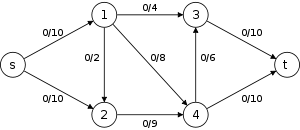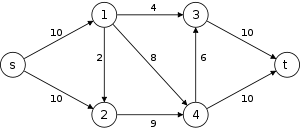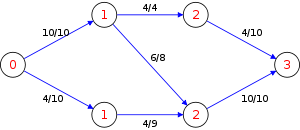The blocking flow consists of

1. {s,1,3,t} with 4 units of flow,
2. {s,1,4,t} with 6 units of flow, and
3. {s,2,4,t} with 4 units of flow.

Therefore the blocking flow is of 14 units and the value of flow | f | is 14. Note that each augmenting path in the blocking flow has 3 edges.

2.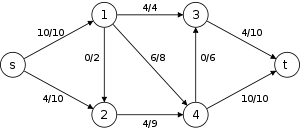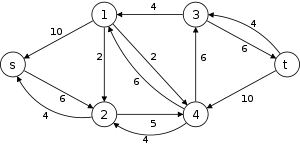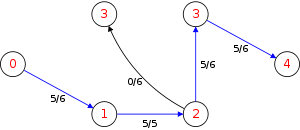The blocking flow consists of

1. {s,2,4,3,t} with 5 units of flow.

Therefore the blocking flow is of 5 units and the value of flow | f | is 14 + 5 = 19. Note that each augmenting path has 4 edges.

3.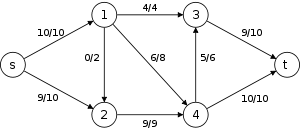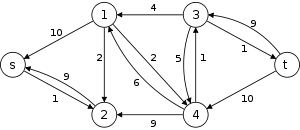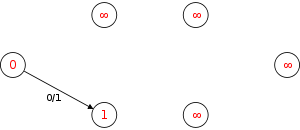Since t cannot be reached in Gf. The algorithm terminates and returns a flow with maximum value of 19. Note that in each blocking flow, the number of edges in the augmenting path increases by at least 1.

## History

Dinic's algorithm was published in 1970 by former Russian Computer Scientist Yefim (Chaim) A. Dinitz , who is today a member of the Computer Science department at Ben-Gurion University of the Negev (Israel), earlier than the Edmonds–Karp algorithm, which was published in 1972 but was discovered earlier. They independently showed that in the Ford–Fulkerson algorithm, if each augmenting path is the shortest one, the length of the augmenting paths is non-decreasing.

Wikimedia Foundation. 2010.

### Look at other dictionaries:

• Edmonds-Karp algorithm — In computer science and graph theory, the Edmonds Karp algorithm is an implementation of the Ford Fulkerson method for computing the maximum flow in a flow network in mathcal{O}(|V| cdot |E|^2). It is asymptotically slower than the relabel to… …   Wikipedia

• Algorithmus von Dinic — Der Algorithmus von Dinic ist ein Algorithmus aus der Graphentheorie zur Bestimmung eines maximalen s t Flusses in einem Netzwerk. Er wurde von E. A. Dinic (Jefim (Chaim) Dinic) entwickelt und 1970 publiziert. Er ist eine Weiterentwicklung des… …   Deutsch Wikipedia

• Criss-cross algorithm — This article is about an algorithm for mathematical optimization. For the naming of chemicals, see crisscross method. The criss cross algorithm visits all 8 corners of the Klee–Minty cube in the worst case. It visits 3 additional… …   Wikipedia

• Levenberg–Marquardt algorithm — In mathematics and computing, the Levenberg–Marquardt algorithm (LMA) provides a numerical solution to the problem of minimizing a function, generally nonlinear, over a space of parameters of the function. These minimization problems arise… …   Wikipedia

• Gauss–Newton algorithm — The Gauss–Newton algorithm is a method used to solve non linear least squares problems. It can be seen as a modification of Newton s method for finding a minimum of a function. Unlike Newton s method, the Gauss–Newton algorithm can only be used… …   Wikipedia

• Maximum flow problem — An example of a flow network with a maximum flow. The source is s, and the sink t. The numbers denote flow and capacity. In optimization theory, the maximum flow problem is to find a feasible flow through a single source, single sink flow network …   Wikipedia

• Алгоритм Диница — полиномиальный алгоритм для нахождения максимального потока в транспортной сети, предложенный в 1970 году израильским (бывшим русским) учёным Ефимом Диницем. Временная сложность алгоритма составляет . Получить такую оценку позволяет введение… …   Википедия

• Method of Four Russians — In computer science, the Method of Four Russians is a technique for speeding up algorithms involving Boolean matrices, or more generally algorithms involving matrices in which each cell may take on only a bounded number of possible values.… …   Wikipedia

• Flüsse und Schnitte in Netzwerken — sind Strukturen der Graphentheorie, die vielfältige Anwendungen finden. Inhaltsverzeichnis 1 Definitionen, wichtige Begriffe und Eigenschaften 1.1 Netzwerk 1.2 Fluss 1.2.1 …   Deutsch Wikipedia

• Linear programming — (LP, or linear optimization) is a mathematical method for determining a way to achieve the best outcome (such as maximum profit or lowest cost) in a given mathematical model for some list of requirements represented as linear relationships.… …   Wikipedia